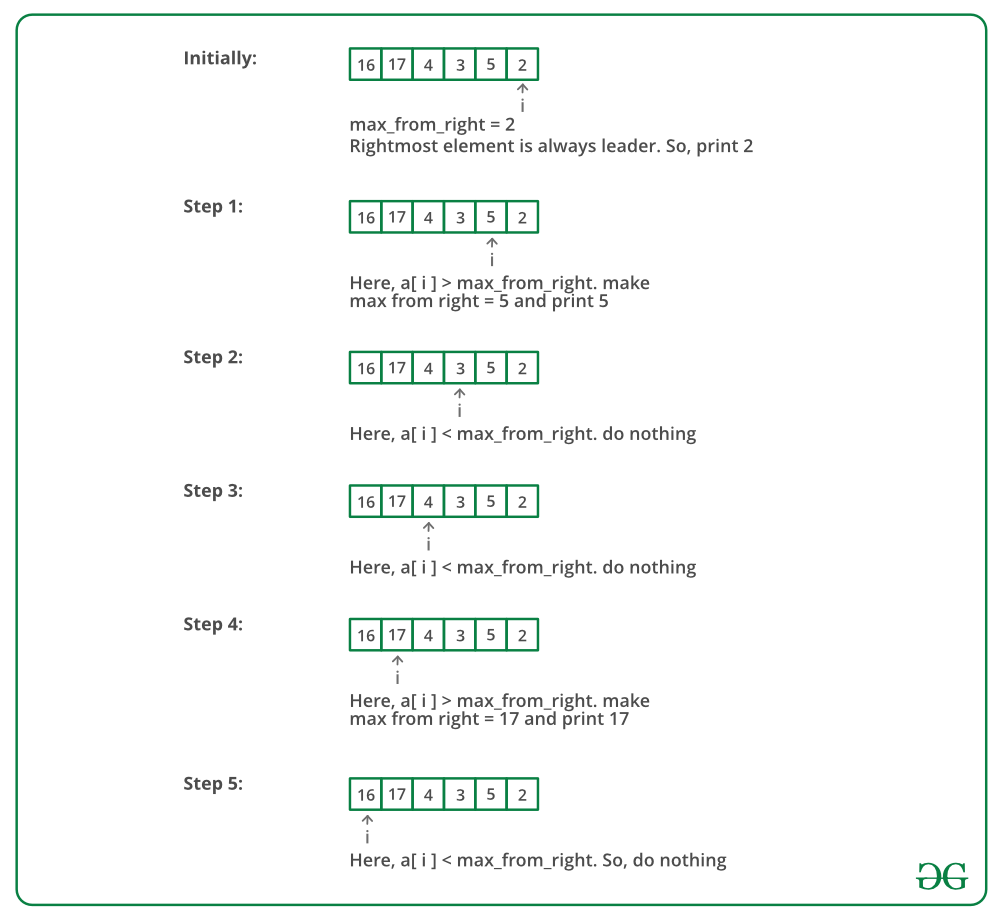• Difficulty Level : Easy
• Last Updated : 30 Jun, 2021

Write a program to print all the LEADERS in the array. An element is leader if it is greater than all the elements to its right side. And the rightmost element is always a leader. For example int the array {16, 17, 4, 3, 5, 2}, leaders are 17, 5 and 2.
Let the input array be arr[] and size of the array be size.

Method 1 (Simple)
Use two loops. The outer loop runs from 0 to size – 1 and one by one picks all elements from left to right. The inner loop compares the picked element to all the elements to its right side. If the picked element is greater than all the elements to its right side, then the picked element is the leader.

Attention reader! Don’t stop learning now. Get hold of all the important DSA concepts with the DSA Self Paced Course at a student-friendly price and become industry ready.  To complete your preparation from learning a language to DS Algo and many more,  please refer Complete Interview Preparation Course.

In case you wish to attend live classes with experts, please refer DSA Live Classes for Working Professionals and Competitive Programming Live for Students.

## C++

 `#include``using` `namespace` `std;` `/*C++ Function to print leaders in an array */``void` `printLeaders(``int` `arr[], ``int` `size)``{``    ``for` `(``int` `i = 0; i < size; i++)``    ``{``        ``int` `j;``        ``for` `(j = i+1; j < size; j++)``        ``{``            ``if` `(arr[i] <=arr[j])``                ``break``;``        ``}   ``        ``if` `(j == size) ``// the loop didn't break``            ``cout << arr[i] << ``" "``;``  ``}``}` `/* Driver program to test above function */``int` `main()``{``    ``int` `arr[] = {16, 17, 4, 3, 5, 2};``    ``int` `n = ``sizeof``(arr)/``sizeof``(arr);``    ``printLeaders(arr, n);``    ``return` `0;``}`

## Java

 `class` `LeadersInArray``{``    ``/*Java Function to print leaders in an array */``    ``void` `printLeaders(``int` `arr[], ``int` `size)``    ``{``        ``for` `(``int` `i = ``0``; i < size; i++)``        ``{``            ``int` `j;``            ``for` `(j = i + ``1``; j < size; j++)``            ``{``                ``if` `(arr[i] <=arr[j])``                    ``break``;``            ``}``            ``if` `(j == size) ``// the loop didn't break``                ``System.out.print(arr[i] + ``" "``);``        ``}``    ``}` `    ``/* Driver program to test above functions */``    ``public` `static` `void` `main(String[] args)``    ``{``        ``LeadersInArray lead = ``new` `LeadersInArray();``        ``int` `arr[] = ``new` `int``[]{``16``, ``17``, ``4``, ``3``, ``5``, ``2``};``        ``int` `n = arr.length;``        ``lead.printLeaders(arr, n);``    ``}``}`

## Python

 `# Python Function to print leaders in array` `def` `printLeaders(arr,size):``    ` `    ``for` `i ``in` `range``(``0``, size):``        ``for` `j ``in` `range``(i``+``1``, size):``            ``if` `arr[i]<``=``arr[j]:``                ``break``        ``if` `j ``=``=` `size``-``1``: ``# If loop didn't break``            ``print` `arr[i],` `# Driver function``arr``=``[``16``, ``17``, ``4``, ``3``, ``5``, ``2``]``printLeaders(arr, ``len``(arr))` `# This code is contributed by _Devesh Agrawal__`

## C#

 `// C# program to print``// leaders in array``using` `System;``class` `GFG``{``    ``void` `printLeaders(``int` `[]arr,``                      ``int` `size)``    ``{``        ``for` `(``int` `i = 0; i < size; i++)``        ``{``            ``int` `j;``            ``for` `(j = i + 1; j < size; j++)``            ``{``                ``if` `(arr[i] <=arr[j])``                    ``break``;``            ``}``            ` `            ``// the loop didn't break``            ``if` `(j == size)``                ``Console.Write(arr[i] + ``" "``);``        ``}``    ``}` `    ``// Driver Code``    ``public` `static` `void` `Main()``    ``{``        ``GFG lead = ``new` `GFG();``        ``int` `[]arr = ``new` `int``[]{16, 17, 4, 3, 5, 2};``        ``int` `n = arr.Length;``        ``lead.printLeaders(arr, n);``    ``}``}` `// This code is contributed by``// Akanksha Rai(Abby_akku)`

## PHP

 ``

## Javascript

 ``

Output:

`17 5 2`

Time Complexity: O(n*n)
Method 2 (Scan from right)
Scan all the elements from right to left in an array and keep track of maximum till now. When maximum changes its value, print it.
Below image is a dry run of the above approach:Below is the implementation of the above approach:

## C++

 `#include ``using` `namespace` `std;` `/* C++ Function to print leaders in an array */``void` `printLeaders(``int` `arr[], ``int` `size)``{``    ``int` `max_from_right =  arr[size-1];` `    ``/* Rightmost element is always leader */``    ``cout << max_from_right << ``" "``;``    ` `    ``for` `(``int` `i = size-2; i >= 0; i--)``    ``{``        ``if` `(max_from_right < arr[i])``        ``{          ``            ``max_from_right = arr[i];``            ``cout << max_from_right << ``" "``;``        ``}``    ``}   ``}` `/* Driver program to test above function*/``int` `main()``{``    ``int` `arr[] = {16, 17, 4, 3, 5, 2};``    ``int` `n = ``sizeof``(arr)/``sizeof``(arr);``    ``printLeaders(arr, n);``    ``return` `0;``}   `

## Java

 `class` `LeadersInArray``{``    ``/* Java Function to print leaders in an array */``    ``void` `printLeaders(``int` `arr[], ``int` `size)``    ``{``        ``int` `max_from_right =  arr[size-``1``];`` ` `        ``/* Rightmost element is always leader */``        ``System.out.print(max_from_right + ``" "``);``     ` `        ``for` `(``int` `i = size-``2``; i >= ``0``; i--)``        ``{``            ``if` `(max_from_right < arr[i])``            ``{          ``            ``max_from_right = arr[i];``            ``System.out.print(max_from_right + ``" "``);``            ``}``        ``}   ``    ``}` `    ``/* Driver program to test above functions */``    ``public` `static` `void` `main(String[] args)``    ``{``        ``LeadersInArray lead = ``new` `LeadersInArray();``        ``int` `arr[] = ``new` `int``[]{``16``, ``17``, ``4``, ``3``, ``5``, ``2``};``        ``int` `n = arr.length;``        ``lead.printLeaders(arr, n);``    ``}``}`

## Python

 `# Python function to print leaders in array``def` `printLeaders(arr, size):``   ` `    ``max_from_right ``=` `arr[size``-``1``]  ``    ``print` `max_from_right,   ``    ``for` `i ``in` `range``( size``-``2``, ``-``1``, ``-``1``):       ``        ``if` `max_from_right < arr[i]:       ``            ``print` `arr[i],``            ``max_from_right ``=` `arr[i]``        ` `# Driver function``arr ``=` `[``16``, ``17``, ``4``, ``3``, ``5``, ``2``]``printLeaders(arr, ``len``(arr))` `# This code contributed by _Devesh Agrawal__`

## C#

 `// C# program to find Leaders in an array``using` `System;` `class` `LeadersInArray {``    ` `    ``// C# Function to print leaders``    ``// in an array``    ``void` `printLeaders(``int` `[]arr, ``int` `size)``    ``{``        ``int` `max_from_right = arr[size - 1];` `        ``// Rightmost element is always leader``        ``Console.Write(max_from_right +``" "``);``    ` `        ``for` `(``int` `i = size - 2; i >= 0; i--)``        ``{``            ``if` `(max_from_right < arr[i])   ``            ``{    ``                ``max_from_right = arr[i];``                ``Console.Write(max_from_right +``" "``);``            ``}``        ``}``    ``}` `    ``// Driver Code``    ``public` `static` `void` `Main(String[] args)``    ``{``        ``LeadersInArray lead = ``new` `LeadersInArray();``        ``int` `[]arr = ``new` `int``[]{16, 17, 4, 3, 5, 2};``        ``int` `n = arr.Length;``        ``lead.printLeaders(arr, n);``    ``}``}` `// This code is contributed``// by Akanksha Rai(Abby_akku)`

## PHP

 `= 0; ``\$i``--)``    ``{``        ``if` `(``\$max_from_right` `< ``\$arr``[``\$i``])   ``        ``{        ``            ``\$max_from_right` `= ``\$arr``[``\$i``];``            ``echo``(``\$max_from_right``);``            ``echo``(``" "``);``        ``}``    ``}``}` `// Driver Code``\$arr` `= ``array``(16, 17, 4, 3, 5, 2);``\$n` `= sizeof(``\$arr``);``printLeaders(``\$arr``, ``\$n``);` `// This code is contributed``// by Shivi_Aggarwal``?>`

## Javascript

 ``

Output:

`2 5 17`

Time Complexity: O(n)